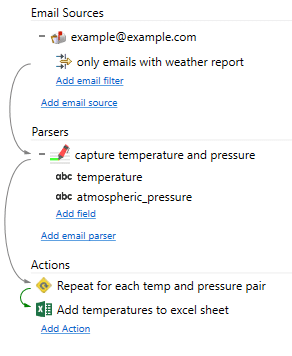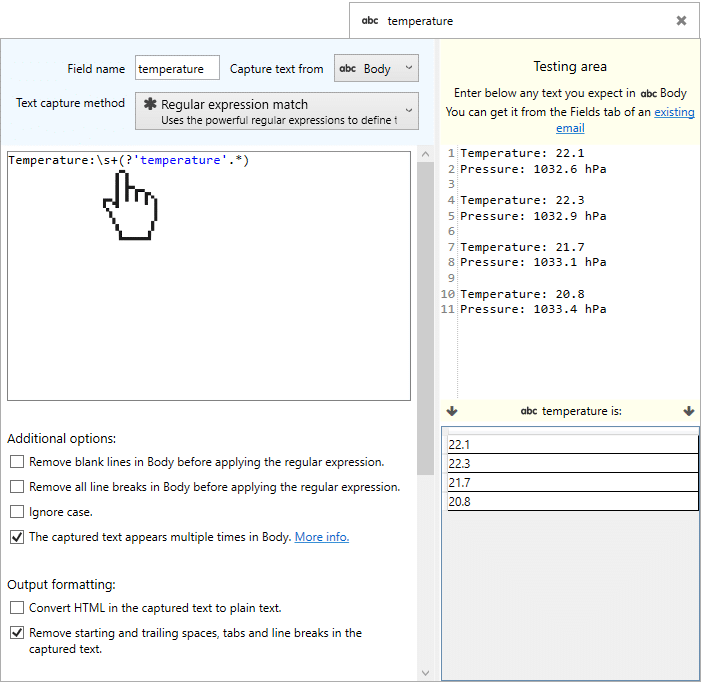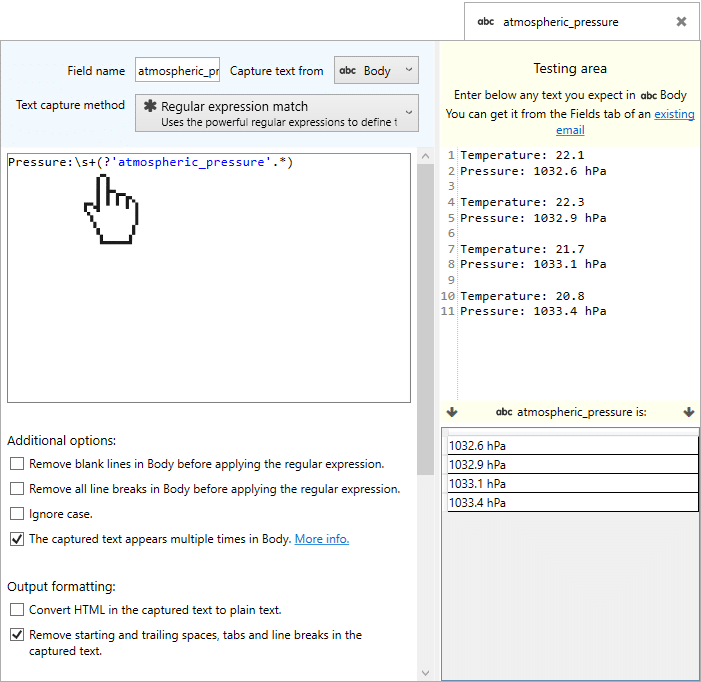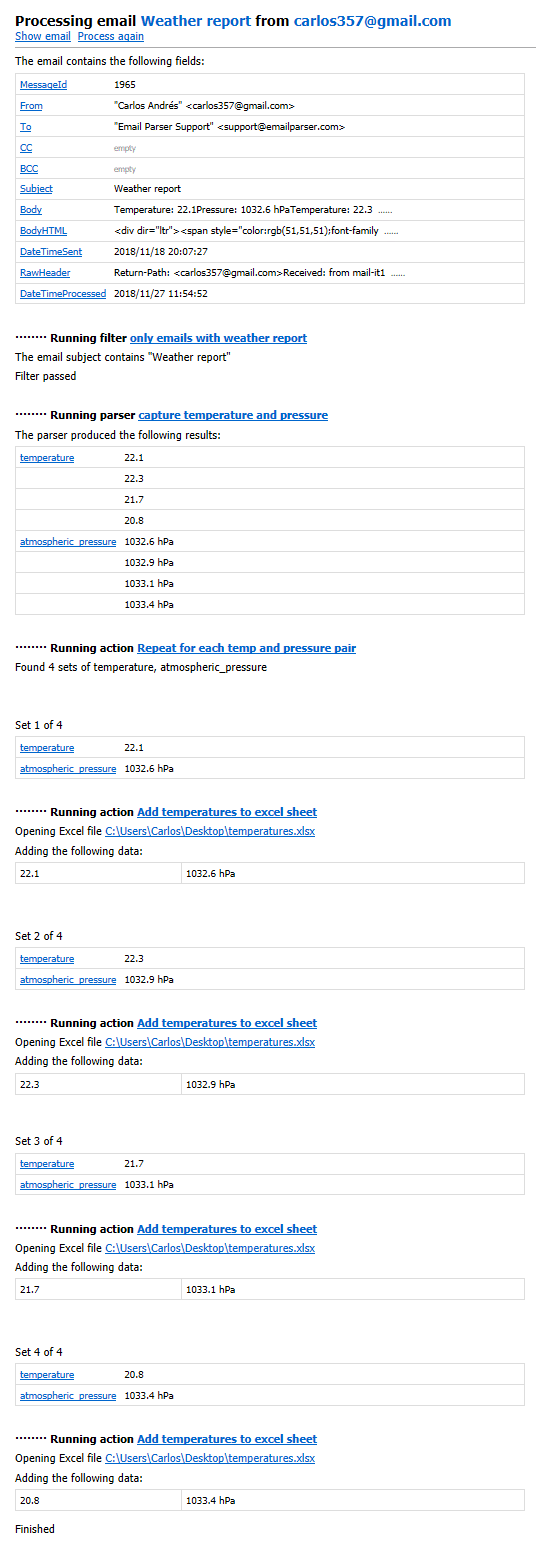Email Parser

Basic regular expression useThis example is based on this previous example but instead of using the text capture method “starts with… continues until…”, which is the most simple one, it uses regular expressions.

The input email is like the following:

 From: test@example.com To: example@example.com Subject: Weather report Temperature: 22.1 Pressure: 1032.6 hPa Temperature: 22.3 Pressure: 1032.9 hPa Temperature: 21.7 Pressure: 1033.1 hPa Temperature: 20.8 Pressure: 1033.4 hPa

In order to capture the temperature and field values we use these regular expressions:For instance, the meaning of the regular expression Temperature:\s+(?’temperature’.*) is the following:

 Regular expression Meaning Temperature:\s+ The word ‘Temperature’ followed by ‘:’ and then followed by one or multiple spaces (?’temperature’.*) The field called ‘temperature’ is any string of consecutive characters.

The output of this example is exactly the same as previous example: